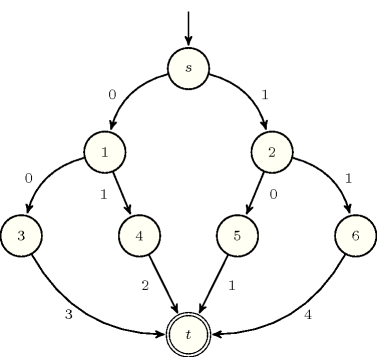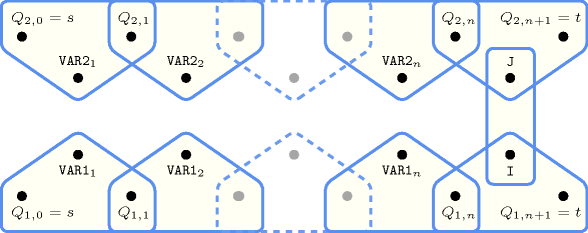## 5.82. cond_lex_greatereq

Origin

Inspired by [WallaceWilson06].

Constraint

$\mathrm{𝚌𝚘𝚗𝚍}_\mathrm{𝚕𝚎𝚡}_\mathrm{𝚐𝚛𝚎𝚊𝚝𝚎𝚛𝚎𝚚}\left(\mathrm{𝚅𝙴𝙲𝚃𝙾𝚁}\mathtt{1},\mathrm{𝚅𝙴𝙲𝚃𝙾𝚁}\mathtt{2},\mathrm{𝙿𝚁𝙴𝙵𝙴𝚁𝙴𝙽𝙲𝙴}_\mathrm{𝚃𝙰𝙱𝙻𝙴}\right)$

Type
 $\mathrm{𝚃𝚄𝙿𝙻𝙴}_\mathrm{𝙾𝙵}_\mathrm{𝚅𝙰𝙻𝚂}$ $\mathrm{𝚌𝚘𝚕𝚕𝚎𝚌𝚝𝚒𝚘𝚗}\left(\mathrm{𝚟𝚊𝚕}-\mathrm{𝚒𝚗𝚝}\right)$
Arguments
 $\mathrm{𝚅𝙴𝙲𝚃𝙾𝚁}\mathtt{1}$ $\mathrm{𝚌𝚘𝚕𝚕𝚎𝚌𝚝𝚒𝚘𝚗}\left(\mathrm{𝚟𝚊𝚛}-\mathrm{𝚍𝚟𝚊𝚛}\right)$ $\mathrm{𝚅𝙴𝙲𝚃𝙾𝚁}\mathtt{2}$ $\mathrm{𝚌𝚘𝚕𝚕𝚎𝚌𝚝𝚒𝚘𝚗}\left(\mathrm{𝚟𝚊𝚛}-\mathrm{𝚍𝚟𝚊𝚛}\right)$ $\mathrm{𝙿𝚁𝙴𝙵𝙴𝚁𝙴𝙽𝙲𝙴}_\mathrm{𝚃𝙰𝙱𝙻𝙴}$ $\mathrm{𝚌𝚘𝚕𝚕𝚎𝚌𝚝𝚒𝚘𝚗}\left(\mathrm{𝚝𝚞𝚙𝚕𝚎}-\mathrm{𝚃𝚄𝙿𝙻𝙴}_\mathrm{𝙾𝙵}_\mathrm{𝚅𝙰𝙻𝚂}\right)$
Restrictions
 $|\mathrm{𝚃𝚄𝙿𝙻𝙴}_\mathrm{𝙾𝙵}_\mathrm{𝚅𝙰𝙻𝚂}|\ge 1$ $\mathrm{𝚛𝚎𝚚𝚞𝚒𝚛𝚎𝚍}$$\left(\mathrm{𝚃𝚄𝙿𝙻𝙴}_\mathrm{𝙾𝙵}_\mathrm{𝚅𝙰𝙻𝚂},\mathrm{𝚟𝚊𝚕}\right)$ $\mathrm{𝚛𝚎𝚚𝚞𝚒𝚛𝚎𝚍}$$\left(\mathrm{𝚅𝙴𝙲𝚃𝙾𝚁}\mathtt{1},\mathrm{𝚟𝚊𝚛}\right)$ $\mathrm{𝚛𝚎𝚚𝚞𝚒𝚛𝚎𝚍}$$\left(\mathrm{𝚅𝙴𝙲𝚃𝙾𝚁}\mathtt{2},\mathrm{𝚟𝚊𝚛}\right)$ $|\mathrm{𝚅𝙴𝙲𝚃𝙾𝚁}\mathtt{1}|=|\mathrm{𝚅𝙴𝙲𝚃𝙾𝚁}\mathtt{2}|$ $|\mathrm{𝚅𝙴𝙲𝚃𝙾𝚁}\mathtt{1}|=|\mathrm{𝚃𝚄𝙿𝙻𝙴}_\mathrm{𝙾𝙵}_\mathrm{𝚅𝙰𝙻𝚂}|$ $\mathrm{𝚛𝚎𝚚𝚞𝚒𝚛𝚎𝚍}$$\left(\mathrm{𝙿𝚁𝙴𝙵𝙴𝚁𝙴𝙽𝙲𝙴}_\mathrm{𝚃𝙰𝙱𝙻𝙴},\mathrm{𝚝𝚞𝚙𝚕𝚎}\right)$ $\mathrm{𝚜𝚊𝚖𝚎}_\mathrm{𝚜𝚒𝚣𝚎}$$\left(\mathrm{𝙿𝚁𝙴𝙵𝙴𝚁𝙴𝙽𝙲𝙴}_\mathrm{𝚃𝙰𝙱𝙻𝙴},\mathrm{𝚝𝚞𝚙𝚕𝚎}\right)$ $\mathrm{𝚍𝚒𝚜𝚝𝚒𝚗𝚌𝚝}$$\left(\mathrm{𝙿𝚁𝙴𝙵𝙴𝚁𝙴𝙽𝙲𝙴}_\mathrm{𝚃𝙰𝙱𝙻𝙴},\left[\right]\right)$ $\mathrm{𝚒𝚗}_\mathrm{𝚛𝚎𝚕𝚊𝚝𝚒𝚘𝚗}$$\left(\mathrm{𝚅𝙴𝙲𝚃𝙾𝚁}\mathtt{1},\mathrm{𝙿𝚁𝙴𝙵𝙴𝚁𝙴𝙽𝙲𝙴}_\mathrm{𝚃𝙰𝙱𝙻𝙴}\right)$ $\mathrm{𝚒𝚗}_\mathrm{𝚛𝚎𝚕𝚊𝚝𝚒𝚘𝚗}$$\left(\mathrm{𝚅𝙴𝙲𝚃𝙾𝚁}\mathtt{2},\mathrm{𝙿𝚁𝙴𝙵𝙴𝚁𝙴𝙽𝙲𝙴}_\mathrm{𝚃𝙰𝙱𝙻𝙴}\right)$
Purpose

$\mathrm{𝚅𝙴𝙲𝚃𝙾𝚁}\mathtt{1}$ and $\mathrm{𝚅𝙴𝙲𝚃𝙾𝚁}\mathtt{2}$ are both assigned to the ${𝙸}^{th}$ and ${𝙹}^{th}$ items of the collection $\mathrm{𝙿𝚁𝙴𝙵𝙴𝚁𝙴𝙽𝙲𝙴}_\mathrm{𝚃𝙰𝙱𝙻𝙴}$ such that $𝙸\ge 𝙹$.

Example
$\left(\begin{array}{c}〈0,0〉,\hfill \\ 〈1,0〉,\hfill \\ 〈\begin{array}{c}\mathrm{𝚝𝚞𝚙𝚕𝚎}-〈1,0〉,\hfill \\ \mathrm{𝚝𝚞𝚙𝚕𝚎}-〈0,1〉,\hfill \\ \mathrm{𝚝𝚞𝚙𝚕𝚎}-〈0,0〉,\hfill \\ \mathrm{𝚝𝚞𝚙𝚕𝚎}-〈1,1〉\hfill \end{array}〉\hfill \end{array}\right)$

The $\mathrm{𝚌𝚘𝚗𝚍}_\mathrm{𝚕𝚎𝚡}_\mathrm{𝚐𝚛𝚎𝚊𝚝𝚎𝚛𝚎𝚚}$ constraint holds since $\mathrm{𝚅𝙴𝙲𝚃𝙾𝚁}\mathtt{1}$ and $\mathrm{𝚅𝙴𝙲𝚃𝙾𝚁}\mathtt{2}$ are respectively assigned to the third and first items of the collection $\mathrm{𝙿𝚁𝙴𝙵𝙴𝚁𝙴𝙽𝙲𝙴}_\mathrm{𝚃𝙰𝙱𝙻𝙴}$.

Typical
 $|\mathrm{𝚃𝚄𝙿𝙻𝙴}_\mathrm{𝙾𝙵}_\mathrm{𝚅𝙰𝙻𝚂}|>1$ $|\mathrm{𝚅𝙴𝙲𝚃𝙾𝚁}\mathtt{1}|>1$ $|\mathrm{𝚅𝙴𝙲𝚃𝙾𝚁}\mathtt{2}|>1$ $|\mathrm{𝙿𝚁𝙴𝙵𝙴𝚁𝙴𝙽𝙲𝙴}_\mathrm{𝚃𝙰𝙱𝙻𝙴}|>1$
Symmetries
• Items of $\mathrm{𝚅𝙴𝙲𝚃𝙾𝚁}\mathtt{1}$, $\mathrm{𝚅𝙴𝙲𝚃𝙾𝚁}\mathtt{2}$ and $\mathrm{𝙿𝚁𝙴𝙵𝙴𝚁𝙴𝙽𝙲𝙴}_\mathrm{𝚃𝙰𝙱𝙻𝙴}.\mathrm{𝚝𝚞𝚙𝚕𝚎}$ are permutable (same permutation used).

• All occurrences of two distinct tuples of values in $\mathrm{𝚅𝙴𝙲𝚃𝙾𝚁}\mathtt{1}$, $\mathrm{𝚅𝙴𝙲𝚃𝙾𝚁}\mathtt{2}$ or $\mathrm{𝙿𝚁𝙴𝙵𝙴𝚁𝙴𝙽𝙲𝙴}_\mathrm{𝚃𝙰𝙱𝙻𝙴}.\mathrm{𝚝𝚞𝚙𝚕𝚎}$ can be swapped; all occurrences of a tuple of values in $\mathrm{𝚅𝙴𝙲𝚃𝙾𝚁}\mathtt{1}$, $\mathrm{𝚅𝙴𝙲𝚃𝙾𝚁}\mathtt{2}$ or $\mathrm{𝙿𝚁𝙴𝙵𝙴𝚁𝙴𝙽𝙲𝙴}_\mathrm{𝚃𝙰𝙱𝙻𝙴}.\mathrm{𝚝𝚞𝚙𝚕𝚎}$ can be renamed to any unused tuple of values.

Usage
Figure 5.82.1 depicts the automaton associated with the preference table of the $\mathrm{𝚌𝚘𝚗𝚍}_\mathrm{𝚕𝚎𝚡}_\mathrm{𝚐𝚛𝚎𝚊𝚝𝚎𝚛𝚎𝚚}$ constraint given in the example. Let $\mathrm{𝚅𝙰𝚁}{\mathtt{1}}_{k}$ and $\mathrm{𝚅𝙰𝚁}{\mathtt{2}}_{k}$ respectively be the $\mathrm{𝚟𝚊𝚛}$ attributes of the ${k}^{th}$ items of the $\mathrm{𝚅𝙴𝙲𝚃𝙾𝚁}\mathtt{1}$ and the $\mathrm{𝚅𝙴𝙲𝚃𝙾𝚁}\mathtt{2}$ collections. Figure 5.82.2 depicts the reformulation of the $\mathrm{𝚌𝚘𝚗𝚍}_\mathrm{𝚕𝚎𝚡}_\mathrm{𝚐𝚛𝚎𝚊𝚝𝚎𝚛𝚎𝚚}$ constraint. This reformulation uses:
• Two occurrences of the automaton depicted by Figure 5.82.1 for computing the positions $𝙸$ and $𝙹$ within the preference table corresponding to $\mathrm{𝚅𝙴𝙲𝚃𝙾𝚁}\mathtt{1}$ and $\mathrm{𝚅𝙴𝙲𝚃𝙾𝚁}\mathtt{2}$.
• The binary constraint $𝙸\ge 𝙹$.
##### Figure 5.82.1. Automaton associated with the preference table of the $\mathrm{𝚌𝚘𝚗𝚍}_\mathrm{𝚕𝚎𝚡}_\mathrm{𝚐𝚛𝚎𝚊𝚝𝚎𝚛𝚎𝚚}$ constraint given in the Example slot##### Figure 5.82.2. Hypergraph of the reformulation corresponding to the $\mathrm{𝚌𝚘𝚗𝚍}_\mathrm{𝚕𝚎𝚡}_\mathrm{𝚐𝚛𝚎𝚊𝚝𝚎𝚛𝚎𝚚}$ constraint: it uses two occurrences of the automaton of Figure 5.82.1 and the constraint $𝙸\ge 𝙹$